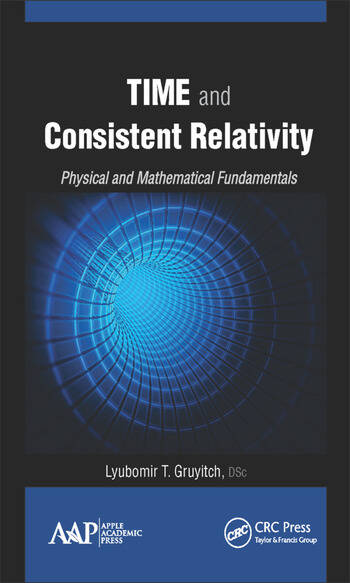Time and Consistent Relativity: Physical and Mathematical Fundamentals

1st Edition

Lyubomir T. Gruyitch

Published May 8, 2015
Reference - 601 Pages - 25 B/W Illustrations
ISBN 9781771881111 - CAT# N11254

USD\$220.00

FREE Standard Shipping!

Preview

Summary

Time and Consistent Relativity: Physical and Mathematical Fundamentals establishes a new and original theory of time relativity, which is fully consistent. It explains why Einstein’s theory of time relativity is physically meaningless and mathematically based on tacit inacceptable assumptions, and why it represents the singular case from the mathematical point of view. The consistent relativity theory established in the book represents an exit from the situation created by Einstein’s theory of time relativity.

This original book presents novel results on time and its relativity that constitute the consistent relativity theory. The results are free of mistakes, inconsistencies, and paradoxes of Einstein’s time relativity theory. The author’s original discoveries and results constitute the new physical and mathematical fundamentals on time and its relativity. The book presentation is concise, clear, and self-contained. It covers the phenomenon of time and its properties and results in a definition and characterization of time. It enables the great variety of new mathematical results presented in the form of theorems and their corollaries and specifies the necessary and sufficient conditions for the corresponding statements to hold. The proofs are rigorous.

This book:

• Explains the physical nature of time
• Presents the definition and characterization of time
• Explains the physical sense of time relativity
• Rejects Einstein’s time relativity theory as the general one
• Proves that Einstein’s time relativity theory represents a singular case valid under tacit, physically meaningless and mathematically inacceptable, assumptions
• Generalizes and extends the Galilean-Newtonian meaning of time and its relativity
• Introduces various new classes of mathematical transformations related to temporal, spatial, and velocity coordinates and proves the necessary and sufficient conditions for their validity
• Discovers a great specter of new results on the time uniqueness, relativity, and temporal speed
• Discovers and proves a great specter of new results on the velocity and its transformations
• Discovers and proves a great specter of new results on the light speed and its invariance and non-invariance
• Discovers and proves the relationship of the light speed and the upper limiting speed
• Opens new directions for further research in physics and mathematical physics